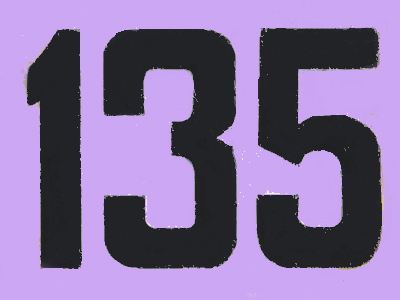The next odd number after 133 is 135.

# Number Sequences 2

This Math quiz is called 'Number Sequences 2' and it has been written by teachers to help you if you are studying the subject at elementary school. Playing educational quizzes is an enjoyable way to learn if you are in the 3rd, 4th or 5th grade - aged 8 to 11.

It costs only \$12.50 per month to play this quiz and over 3,500 others that help you with your school work. You can subscribe on the page at Join Us

Recognizing patterns in number sequences is all about finding out what comes next in a row of numbers. For instance, do you know which number should follow this sequence 20, 15, 10, 5? Well, the numbers are decreasing. or getting less, each time. Each number is 5 less than the one before it. To find the next number in the sequence we need to take 5 away from 5 which will give us the answer 0.

1.
Which of these statements is true?
After 1, every third number is even
After 1, every second number is odd
Every second number starting at 1 is odd.
Every second number starting at 2 is even
2.
Can you find the rule of this sequence? 45, 35, 25, 15, 5
The numbers decrease in 2s
The numbers decrease in 3s
The numbers decrease in 5s
The numbers decrease in 10s
Each number (except the first) is 10 less than the one before it
3.
What are the next two numbers of the sequence? 4, 8, 12, 16
18, 20
20, 22
20, 24
20, 26
The numbers are increasing in 4s
4.
Can you find the rule of this sequence? 50, 75, 100, 125, 150
The numbers increase in 20s
The numbers increase in 25s
The numbers increase in 5s
The numbers increase in 10s
Each number (except the first) is 25 more than the one before it
5.
Which is the next odd number after 151?
152
153
155
160
151 + 2 = 153
6.
Which of these statements is true?
An even number ends in 0, 2, 4, 6 or 8
An odd number ends in 0 or 5
An even number ends in 1, 3, 5, 7 or 9
An odd number ends in 0, 2, 4, 6 or 8
Even numbers end in 0, 2, 4, 6 or 8.
Odd numbers end in 1, 3, 5, 7 or 9
7.
Can you find the rule of this sequence? 125, 127, 129, 131, 133
The numbers increase in 2s
The numbers increase in 3s
The numbers increase in 5s
The numbers increase in 4s
The sequence would continue: 135, 137, 139, 141... etc
8.
Can you find the rule of this sequence? 5, 8, 11, 14, 17
The numbers increase in 2s
The numbers increase in 3s
The numbers increase in 5s
The numbers increase in 10s
Each number (except the first) is 3 more than the one before it
9.
What is the next number of the sequence? 136, 140, 144, 148
150
152
154
156
The numbers are increasing in 4s
10.
What is the next number of the sequence? 140, 130, 120, 110
90
95
100
105
The numbers are decreasing by 10
Author:  Amanda Swift